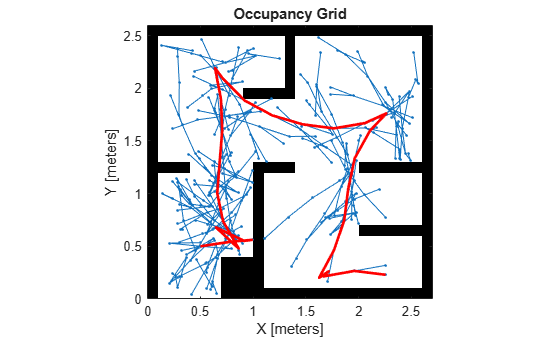Documentation

# stateSpaceReedsShepp

State space for Reeds-Shepp vehicles

## Description

The `stateSpaceReedsShepp` object stores parameters and states in the ReedsShepp state space, which is composed of state vectors represented by [x, y, θ]. x and y are Cartesian coordinates, and θ is the orientation angle. The Reeds-Shepp state space has a lower limit on the turning radius (specified by the `MinTurningRadius` property in the object) and forward and reverse costs (specified by the `ForwardCost` and `ReverseCost` properties in the object) for navigating between states.

## Creation

### Syntax

``space = stateSpaceReedsShepp``
``sapce = stateSpaceReedsShepp(bounds)``

### Description

````space = stateSpaceReedsShepp` creates a Reeds-Shepp state space object with default state bounds for x, y, and θ. ```
````sapce = stateSpaceReedsShepp(bounds)` specifies the `bounds` for x, y, and θ. The state values beyond the bounds are truncated to the bounds. The input, `bounds`, sets the value of the StateBounds property.```

## Properties

expand all

Name of state space, specified as a string.

Dimension of the state space, specified as a positive integer.

Bounds of state variables, specified as a 3-by-2 real-valued matrix.

• The first row specifies the lower and upper bounds for the x state in meters.

• The second row specifies the lower and upper bounds for the y state in meters.

• The third row specifies the lower and upper bounds for the θ state in radians.

Data Types: `double`

Minimum turning radius in meters, specified as a positive scalar. The minimum turning radius is for the smallest circle the vehicle can make with maximum steer in a single direction.

Cost multiplier for forward motion, specified as a positive scalar. Increase the cost to penalize forward motion.

Cost multiplier for reverse motion, specified as a positive scalar. Increase the cost to penalize reverse motion.

## Object Functions

 `copy` Create copy of state space object `distance` Distance between two states `enforceStateBounds` Ensure state lie within state bounds `interpolate` Interpolate between states `sampleGaussian` Sample state using Gaussian distribution `sampleUniform` Sample state using uniform distribution

## Examples

collapse all

Create a ReedsShepp state space.

`ss = stateSpaceReedsShepp;`

Create an `occupanyMap`-based state validator using the created state space.

`sv = validatorOccupancyMap(ss);`

Create an occupany map from an example map and and set map resolution as 10 cells/meter.

```load exampleMaps map = occupancyMap(simpleMap,10); sv.Map = map;```

Set validation distance for the validator.

`sv.ValidationDistance = 0.01;`

Update state space bounds to be the same as map limits.

`ss.StateBounds = [map.XWorldLimits;map.YWorldLimits; [-pi pi]];`

Create the path planner and increase max connection distance.

```planner = plannerRRT(ss,sv); planner.MaxConnectionDistance = 0.3;```

Set the start and goal states.

```start = [0.5,0.5,0]; goal = [2.5,0.2,0];```

Plan a path with default settings.

```rng(100,'twister'); % repeatable result [pthObj,solnInfo] = planner.plan(start,goal);```

Visualize the results.

```show(map); hold on; plot(solnInfo.TreeData(:,1),solnInfo.TreeData(:,2),'.-'); % tree expansion plot(pthObj.States(:,1), pthObj.States(:,2),'r-','LineWidth',2) % draw path```## Extended Capabilities

### C/C++ Code GenerationGenerate C and C++ code using MATLAB® Coder™.